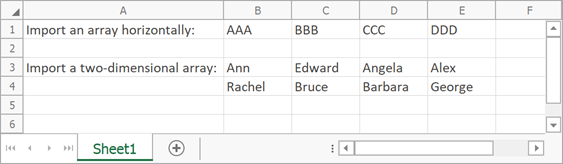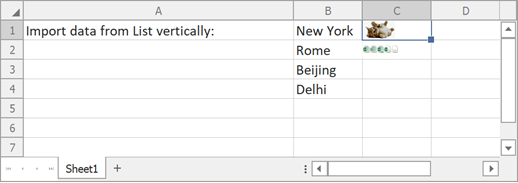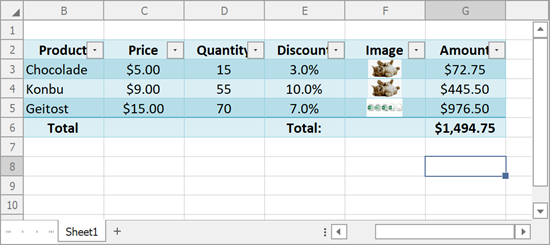21.1
20.2
20.1
19.2
19.1
18.2
18.1
17.2
A newer version of this page is available. Switch to the current version.

# How to: Import Data to a Worksheet

• 4 minutes to read

You can import data to worksheet cells from different data sources (for example, arrays, lists and data tables) via the worksheet’s Import extension method.

Pass the following parameters to the WorksheetExtensions.Import method.

• An object that specifies a source for importing data.
• Row and column indexes of the start cell in which you wish to insert imported data into the worksheet.
• A Boolean value specifying whether to insert imported data vertically or horizontally. Use this parameter when importing data from a one-dimensional array or list.
##### IMPORTANT

Use of this method in production code requires a license to the DevExpress Office File API or the DevExpress Universal Subscription.

Worksheet extensions are defined by the WorksheetExtensions class. To enable them, add a reference to the DevExpress.Docs.v18.2.dll library and explicitly import the DevExpress.Spreadsheet namespace into your source code with a using directive (Imports in Visual Basic).

``````
using DevExpress.Spreadsheet;
``````
##### TIP

If you do not own the Office File API subscription and cannot reference the DevExpress.Docs.v18.2.dll, bind a read-only data source as described in the How to Bind a Spreadsheet to a List of Objects document and subsequently remove the binding using the WorksheetDataBindingCollection.Remove or WorksheetDataBindingCollection.Clear method.

## Import Data from Arrays

``````// Create an array containing string values.
string[] array = new string[] { "AAA", "BBB", "CCC", "DDD" };

// Import the array into the worksheet and insert it horizontally, starting with the B1 cell.
worksheet.Import(array, 0, 1, false);

// Create a two-dimensional array containing string values.
String[,] names = new String[2, 4]{
{"Ann", "Edward", "Angela", "Alex"},
{"Rachel", "Bruce", "Barbara", "George"}
};

// Import the two-dimensional array into the worksheet and insert it, starting with the B3 cell.
worksheet.Import(names, 2, 1);
``````

The image below shows the results.## Import Data from a List

``````// Create a List object containing string values.
List<string> cities = new List<string>();
cities.Add("New York");
cities.Add("Rome");
cities.Add("Beijing");
cities.Add("Delhi");

// Import the list into the worksheet and insert it vertically, starting with the B1 cell.
worksheet.Import(cities, 0, 1, true);
``````

The image below shows the results.## Import Data from a DataTable

The following code illustrates how to import data from a DataTable object to a worksheet. Note that the cell data types are set automatically, according to the data types of the source column. Cell formats are set automatically to the default value for the cell data type. However, you can easily change them, as described in the How to: Specify Number or Date Format for Cell Content topic.

``````void ImportDataTable() {

Worksheet worksheet = spreadsheetControl1.Document.Worksheets;

// Create a "Products" DataTable object with four columns.
DataTable sourceTable = new DataTable("Products");
sourceTable.Columns.Add("Product", typeof(string));
sourceTable.Columns.Add("Price", typeof(float));
sourceTable.Columns.Add("Quantity", typeof(Int32));
sourceTable.Columns.Add("Discount", typeof(float));

sourceTable.Rows.Add("Chocolade", 5, 15, 0.03);
sourceTable.Rows.Add("Konbu", 9, 55, 0.1);
sourceTable.Rows.Add("Geitost", 15, 70, 0.07);

// Import data from the data table into the worksheet and insert it, starting with the B2 cell.
worksheet.Import(sourceTable, true, 1, 1);
}
``````

The image below shows the results.